Frontpage Search Email . Danish .Welcome to my homepage, developing the classic head physics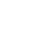Mass formation If you have not put yourself into the system that I use, then understanding the basis for this section is not present. This section is a continuation of the classic Big Bang section.System integration requirementsTo work with physical systems, it is necessary to have a set of physical and mathematical tools.Some of them I pick up directly in classical physics and some I have to develop myself. There are some that cannot be used because they cannot be integrated into a single physical system, eg Niels Bohr's quantum physics and the like. Newton's gravity is ok, but if you come out of the large universe where the parts are merged into a larger whole, it can not be used in the form that Newton used.So there is an integration requirement that must meet and not all physical systems can meet them.The shift in the thermal balance of the universe causes a span which has two velocity vectors, free fall c2 and resting span 1 / c2 (see classic big bang fig. b.36).The vectors have the same value, measured in energy, but must be kept separate in the mathematical system.because the volume from which the energy is calculated does not have the same state. Normally: free fall speed vector m = Vx * c2 = (4/3 * pi * r3) * c2 the volume is taken out from the thermal resting point of the universe.Mass: dormant speed vector mb = Vb * c2 = (4/3 * pi * (1/c2)3) * c2, the volume is taken from the compressed volume. ..................................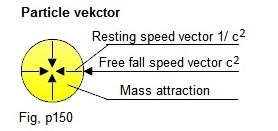If the particle mass is not at rest and is affected by a force, then the vectors and volume must be corrected.With the speed of light, the universe can gather millions of parts to the absolute accuracy. We use a unit-controlled number system, we cannot assemble two parts to the absolute accuracy even though we spend unlimited time on it. This means that it is not possible to assemble the parts of the universe mathematically without also using combination solutions, but it does not change that we need to know how the individual parts are put together.The core of the universe:The universe can only create one particle in the Big Bang process (see classic big bang figure 3.1).I call the particle a basic core. The mass of the basic core is calculated as follows: .............mbasis = ((4/3) * pi *  r3) *  c2 = 5.185681022 * 10-34kg.Many years ago I made a very thorough study of the nucleus of the atom. Here it was found that the energy of the nucleus is stabilized by the binding structure of the nucleons (see Atomic binding Fig. 1.8).The binding structure is the way of the universe to adapt the nuclei to the surrounding space and can be found in almost all structures, including in molecular crystals. ...........................................A proton consists of approx. 3 million basic cores.When a proton gets crushed in a particle accelerator, the weakest joints will collapse first, making all the fragments not fall apart at the same time, but will divide into smaller and smaller pieces. The images coming from the accelerator are completely in line with the system used here. The problem is how to handle it mathematically.Coordinators and Vectors of the Universe:All function vectors in the Universe always refer to the resting point of the universe.It is therefore appropriate to use a coordinate system which indicates the speed vector reference to the 0 point. The volume of the universe is therefore allocated an infinite number of points, the coordinate will look like this next to the 0 point of the universe (0, 0, 0,) ........................................You will typically encounter the problem of emission of radiation in particle velocities and, incidentally, the universe is a huge, cohesive network of vectors that all refer to the 0 point of the universe.When a particle lies still and is not affected by any forces, it will have the shape of a ball.The velocity vector of the particle points to the center, which makes it stand upside down in relation to the normal function of the Universe.FIG. p329 shows two velocity vectors having different length, free fall c2 and compressed 1 / c2. When a particle is applied to kinetic energy, the compressed portion will be displaced and the free fall vector correspondingly reduced, the result being that the particle changes shape. ......................Here I have shown the basic part in partial speed. Underlying is that a particle is made up of basic cores  and here we have the particle's deceleration and the emission function of the radiation.Notes on particle propertiesI just want to show what is to be integrated into the system:There are a number of functions associated with moving particles. Example: ........................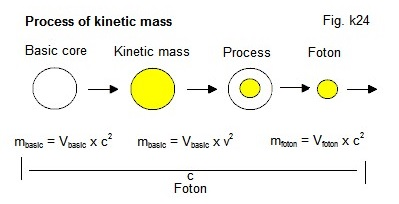You can read more about this in my section about Particle Radiation. Mass and radiation have an action and reaction system as shown here. ...............................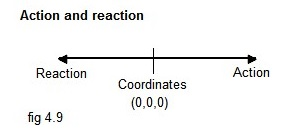The compensation system in the universe: The universe can gather millions of parts to absolute accuracy with the speed of light.We use a unit-controlled system; we cannot assemble two parts to absolute accuracy, even if we spend unlimited time on it. This means that some parts of the universe cannot be assembly with the number system we use, even with computer simulation.It is therefore necessary that some parts of the universe's developmental system must be developed on a system of compensation. ..............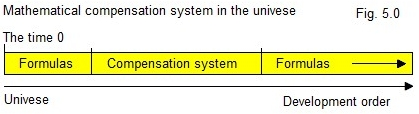The universe has a combined composite development system. The universe uses only some of them. This part cannot be changed (they are locked).There are a number of development parts that the universe does not use and which can be used for technical solutions and more.Summary of formulas and mathematical systems used:   Mass: mx = Vx * c2 = (4/3 * pi * r3) * c2 Basic particle: mb = Vb * c2 = (4/3 * pi * (1/c2)3) * c2 Kinetic mass: mx = Vx * v2 = (4/3 * pi * r3) * v2 Kinetic action and reaction mass: mx = ½ * Vx * v2 = ½ * (4/3 * pi * r3) * v2 Particle mass attraction: mx = (Vx * c2) / V Molecule mass attraction: mx = (Vx * v2) / V Radiation: mbasis2 = (Vbasis * c2) + (Vbasis * dv2 ) mbasis2 = (Vbasis * c2 ) + (Vfoton * c2) (ikke testet) Wave lengths: yx = y1 + y2 + y3 + y4 (ikke testet) Press here:The universe's head formulas. Albert Einstein's formula for resting energy E = m * c2. He has been very good at finding the right formulas, this one hits exactly in the mass and energy of the universe.You can only convert energy in a way in the universe, everything will refer to this system, if it cannot be converted to this system then there is an error.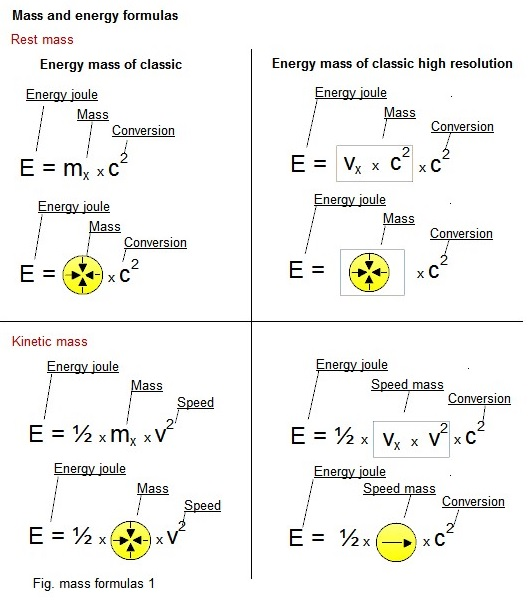Here I show some of the basic systems that are many more.You may want to try making some conversions for these formulas yourself.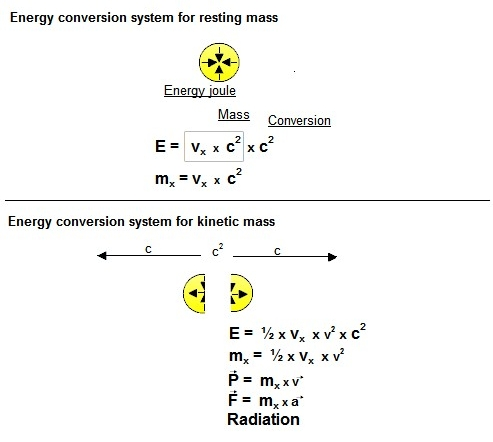Compatible properties between the main function of the universe and known physical systems.A: The system used here is a unified development system in the universe, the parts used must fit into the system.Newton's gravitational system does not.It dates from his Principia of 1687. Time taken a good job into consideration. He could not know anything about the universe having a developmental system.B: Particle vectors are nothing new in the physical world, here we are talking about an expansion within the inner structure of the particles and what a straight line velocity is in the universe and more.C: Temperature measurement systems are available in many variants (mercury, spirits, feathers and electronic thermometers) and are a quick way to measure the thermal state of a molecule.The problem is that it is not a parameter under which the universe function system works.D: There are not many things in the radiation field that work. This is because one has not known that a basic particle exists. The base particle is the building block of all particles and is also the one that absorbs and emits radiation.E: The molecular systems that have been used so far I will call some sort of mess. A molecule is formed from the mass attraction of the particles trying to pull the molecule part together and the radiation pressure trying to push them away from each other.F: You know nothing about what's inside a nuclear nucleus. What you know is what comes out from the core.At this point, I can say that it works very differently than most people had expected.G: A magnetic field consists of several composite parts, which can also function individually and independently.Particle properties between a proton and an electronThe universe mass consists of a two parameter system and there are only two particles that are stable in the rest state (a proton and electron).It is important to know how the universe stabilizes its energy in particles.As you can see here; the electron and proton have many properties in common.The proton has atom # 1 and is part of the atomic nucleus mass and molecular structure, because the base nucleus binding energy locks the nucleus at c2 and is the same as the nucleus resting energy.The electron has a rectilinear c stabilization (the reference point is the resting point of the universe) and does not incorporate directly into any binding structures.There are some holes in the core's binding structure where it can find a resting place, which is why it can be used in electrical conductor, among other things.k Last updated  January 01, 2020 Email : info@jwhdk.euClassic big bang. Mass formation. Atom structure. Atom binding. Molecular binding. Particle radiation Magnetic fields. Mass attraction. Conclusion. Micro physicsUltima update : 1 march 2019
Email : info@jwhdk.eu